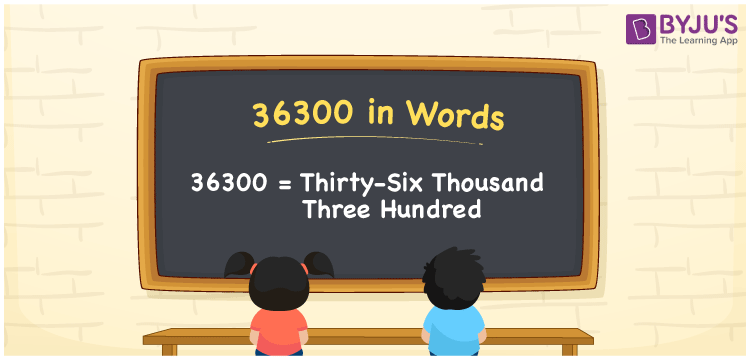# 36300 in Words

Thirty-six Thousand Three Hundred is the number name of 36300. The number 36300 is a natural number, which generally refers to the count or the quantity of things. For example, there are 36300 people in an apartment building. For converting the number 36300 into words, we will employ a place value system. This article will guide you to write the number 36300 in words with a complete explanation.

 36300 in Words: Thirty-six Thousand Three Hundred. Thirty-six Thousand Three Hundred in Numerical Form: 36300.

## 36300 in English Words## How to Write 36300 in Words?

The number 36300 is a natural number and its place values are presented in the below table.

 Ten-thousand Thousands Hundreds Tens Ones 3 6 3 0 0

The expanded form of 36300 is as follows:

= 3 × Ten thousand + 6 × Thousand + 3 × Hundred + 0 × Ten + 0 × One

= 3 × 10000 + 6 × 1000 + 3 × 100 + 0 × Ten + 0 × One

= 30000 + 6000 + 300

= 36300

= thirty-six thousand three hundred

Hence, 36300 in words is thirty-six thousand three hundred.

36300 in words – Thirty-six thousand three hundred

Is 36300 an odd number? – No

Is 36300 an even number? – Yes

Is 36300 a perfect square number? – No

Is 36300 a perfect cube number? – No

Is 36300 a prime number? – No

Is 36300 a composite number? – Yes

## Frequently Asked Questions on 36300 in Words

Q1

### How to spell 36300 in English words?

36300 in words is thirty-six thousand three hundred.

Q2

### Simplify 36000 + 300, and express it in words.

Simplifying 36000 + 300, we get 36300. Hence, 36300 in words is thirty-six thousand three hundred.

Q3

### Is 36300 an odd number?

No, 36300 is not an odd number.